Next: Artificial data Up: tr04mw1 Previous: Classifying SPMs in FMRI

# Inference

To explore the differences that certain aspects of the models make, there are three different mixture models which we want to be able to infer upon in this paper, these are:

• Model 1. Non-spatial with global class proportions (equation 9)
• Model 2. Spatial without adaptive spatial regularisation with fixed spatial smoothness (equation 14 with fixed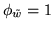)
• Model 3. Spatial with adaptive spatial regularisation (equation 18)
To achieve this we need to obtain the marginal posterior distribution for the weights,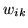, given the observed data,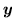. Whilst it is possible to perform approximations to the distribution, it is difficult to assess the effect of these approximations on the inference performed. Therefore, the approach taken instead is to use Markov Chain Monte Carlo (MCMC) sampling from the full joint posterior distribution (see Gilks et al. (1996) and  Gamerman (1997) for texts on MCMC). This also automatically provides us with samples from the marginal posterior distribution for the weights.

We are able to use Gibbs sampling for the adaptive MRF smoothness parameter,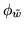. See the appendix for the required full conditional distribution of.

For all other parameters (i.e. the continuous weights and class distribution parameters) we use single-component Metropolis-Hastings jumps (i.e. we propose separate jumps for each of the parameters in turn). The updates are detailed in the appendix. We use separate Normal proposal distributions for each parameter, with the mean fixed on the current value, and with a scale parameter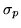for the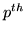parameter that is updated every 30 jumps. At the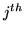updateis updated according to: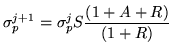(24)

where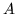and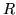are the number of accepted and rejected jumps since the lastupdate respectively,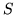is the desired rejection rate, which we fix at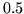.

We require a good initialisation of the parameters in the model purely to reduce the required burn-in of the MCMC chains (the burn-in is the part of the MCMC chain which is used to ensure that the chain has converged to be sampling from the true distribution). To initialise we use the non-spatial class labels with class proportions model (equation 5) along with the class distributions specified in section 3. The joint maximum a posterior over class labels,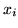, and distribution parameters,, can be obtained for this model using the Expectation-Maximisation (EM) algorithm (Beckmann et al., 2003).

As a result of this good initialisation, we use a burn-in of 1000 jumps, followed by 1000 further jumps of which every 2nd is sampled. Observation of the chains with different initial conditions confirmed that a burn-in of 1000 jumps was sufficient.Next: Artificial data Up: tr04mw1 Previous: Classifying SPMs in FMRI